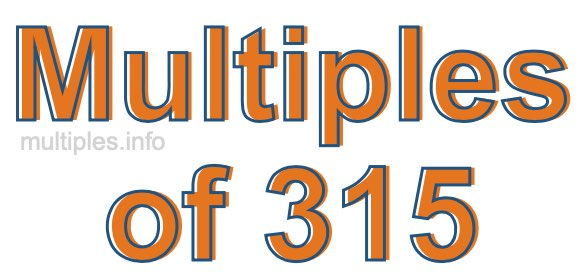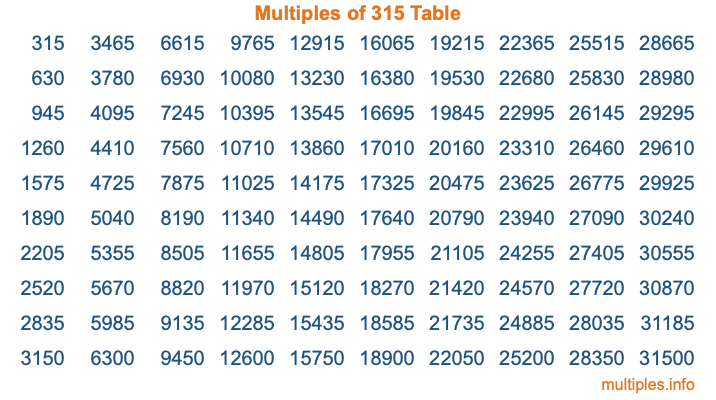Multiples of 315Welcome to the Multiples of 315 page. Here we will first teach you everything you will ever need to know about the multiples of 315, and then give you a study guide summary of everything we taught you to make sure you remember it all. Use this page to look up facts and learn information about the multiples of 315. This page will make you a multiples of three hundred fifteen expert!

Definition of Multiples of 315
Multiples of 315 are all the numbers that when divided by 315 equal an integer. Each of the multiples of 315 are called a multiple. A multiple of 315 is created by multiplying 315 by an integer.

Therefore, to create a list of multiples of 315, you start with 1 multiplied by 315, then 2 multiplied by 315, then 3 multiplied by 315, and so on for as long as you want. Thus, the list of the first five multiples of 315 is 315, 630, 945, 1260, and 1575. To see a larger list of multiples of 315, see the printable image of Multiples of 315 further down on this page. We also have a category where you can choose any nth multiple of 315.

Multiples of 315 Checker
The Multiples of 315 Checker below checks to see if any number of your choice is a multiple of 315. In other words, it checks to see if there is any number (integer) that when multiplied by 315 will equal your number. To do that, we divide your number by 315. If the the quotient is an integer, then your number is a multiple of 315.

Is  a multiple of 315?

Least Common Multiple of 315 and ...
A Least Common Multiple (LCM) is the lowest multiple that two or more numbers have in common. This is also called the smallest common multiple or lowest common multiple and is useful to know when you are adding our subtracting fractions. Enter one or more numbers below (315 is already entered) to find the LCM.

Check out our LCM Calculator if you need more details about the Least Common Multiple or if you need the LCM for different numbers for adding and subtraction fractions.

nth Multiple of 315
As we stated above, 315 is the first multiple of 315, 630 is the second multiple of 315, 945 is the third multiple of 315, and so on. Enter a number below to find the nth multiple of 315.

th multiple of 315

Multiples of 315 vs Factors of 315
315 is a multiple of 315 and a factor of 315, but that is where the similarities end. All postive multiples of 315 are 315 or greater than 315. All positive factors of 315 are 315 or less than 315.

Below is the beginning list of multiples of 315 and the factors of 315 so you can compare:

Multiples of 315: 315, 630, 945, 1260, 1575, etc.

Factors of 315: 1, 3, 5, 7, 9, 15, 21, 35, 45, 63, 105, 315

As you can see, the multiples of 315 are all the numbers that you can divide by 315 to get a whole number. The factors of 315, on the other hand, are all the whole numbers that you can multiply by another whole number to get 315.

It's also interesting to note that if a number (x) is a factor of 315, then 315 will also be a multiple of that number (x).

Multiples of 315 vs Divisors of 315
The divisors of 315 are all the integers that 315 can be divided by evenly. Below is a list of the divisors of 315.

Divisors of 315: 1, 3, 5, 7, 9, 15, 21, 35, 45, 63, 105, 315

The interesting thing to note here is that if you take any multiple of 315 and divide it by a divisor of 315, you will see that the quotient is an integer.

Multiples of 315 Table
Below is an image of the first 100 multiples of 315 in a table. The table is in chronological order, column by column. The first column has the first ten multiples of 315, the second column has the next ten multiples of 315, and so on.The Multiples of 315 Table is also referred to as the 315 Times Table or Times Table of 315. You are welcome to print out our table for your studies.

Negative Multiples of 315
Although not often discussed or needed in math, it is worth mentioning that you can make a list of negative multiples of 315 by multiplying 315 by -1, then by -2, then by -3, and so on, to get the following list of negative multiples of 315:

-315, -630, -945, -1260, -1575, etc.

Multiples of 315 Summary
Below is a summary of important Multiples of 315 facts that we have discussed on this page. To retain the knowledge on this page, we recommend that you read through the summary and explain to yourself or a study partner why they hold true.

There are an infinite number of multiples of 315.

A multiple of 315 divided by 315 will equal a whole number.

315 divided by a factor of 315 equals a divisor of 315.

The nth multiple of 315 is n times 315.

The largest factor of 315 is equal to the first positive multiple of 315.

315 is a multiple of every factor of 315.

315 is a multiple of 315.

A multiple of 315 divided by a divisor of 315 equals an integer.

315 divided by a divisor of 315 equals a factor of 315.

Any integer times 315 will equal a multiple of 315.

Multiples of a Number
Here you can get the multiples of another number, all with the same attention to detail as we did for multiples of 315 on this page.

Multiples of
Multiples of 316
Did you find our page about multiples of three hundred fifteen educational? Do you want more knowledge? Check out the multiples of the next number on our list!